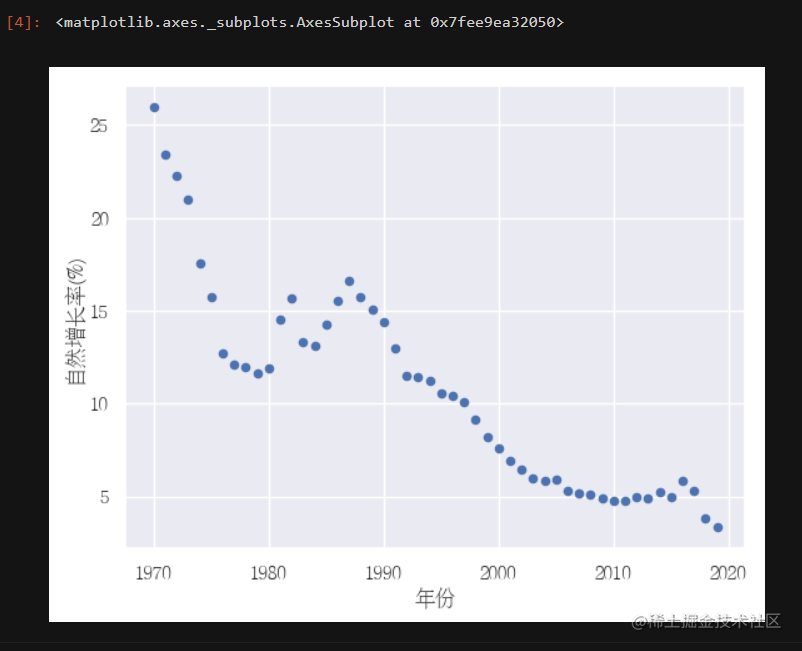# 1. Pandas畫圖

pandas庫中集成了matplotlib庫，可以直接進行呼叫，在相應的DataFrame後，加上.plot就能直接進行繪圖，具體語法格式如下所示：

`pandas.DataFrame.plot`

• DataFrame.plt(x=None, y=None, kind='line')
• x：x資料
• y：y資料
• kind：圖型別

• 'line'：折線圖
• 'bar'：柱狀圖
• 'barth'：
• 'hist'：直方圖
• 'pie'：餅圖
• 'scatter'：散點圖

```python

# 看一下兩者之間的關係x="年份", y="自然增長率(%)"

import pandas as pd import seaborn as sns from pylab import mpl from matplotlib.font_manager import FontProperties myfont=FontProperties(fname=r'/usr/share/fonts/fangzheng/FZSYJW.TTF',size=12) sns.set(font=myfont.get_name())

people = pd.read_csv("data/data140190/人口.csv") people.plot(x="年份", y="自然增長率(%)", kind="scatter") ``` 程式碼執行結果如下圖所示：# 6. 檔案讀取與儲存

• `pandas.read_csv(filepath_or_buffer, sep = ',')`